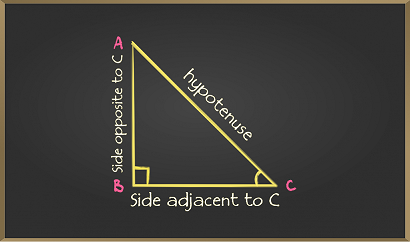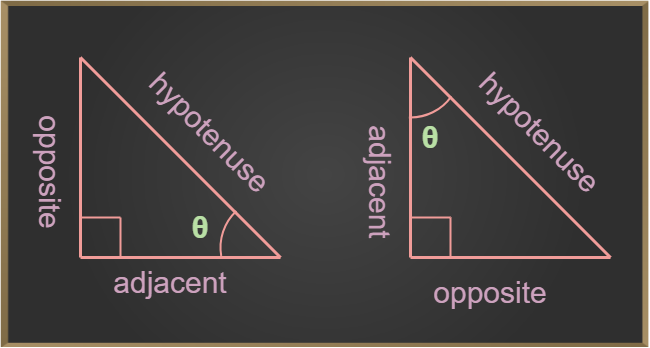Open in App
Not now

# Sin Cos Tan Values

• Last Updated : 08 Dec, 2022

Sin, Cos, and Tan are the basic ratios of Trigonometry that are used to study the relationship between the angles and respective sides of a triangle. These ratios are initially defined on a Right Angled Triangle using Pythagoras Theorem

## What is Sin Cos Tan in Trigonometry?

Let’s understand Sin, Cos, and Tan in trigonometry using formulas and examples.

A triangle that has one angle of 90° is called a right-angled triangle. It has sides called the base, perpendicular (height), and hypotenuse. The right-angled triangle follows the Pythagoras theorem.

• Base: The side which contains the angle is called the base of the triangle.
• Perpendicular (Height): The side which forms 90° with the base is called perpendicular or the height of the triangle.
• Hypotenuse: The longest side of the triangle is called the hypotenuse of the triangle.Sin, Cos, and Tan are the ratios of the sides of any right-angled triangle. In the right-angled triangle ABC given above for angle C the Sin, Cos, and Tan are,

• Sin C = Perpendicular / Hypotenuse = AB / CA
• Cos C = Base / Hypotenuse = BC / CA
• Tan C = Perpendicular / Base = AB / BC

## Sin Cos Tan Values

Sin, Cos, and Tan values are the value of specific angles of a right-angled triangle. In trigonometry, the values of Sin, Cos, and Tan are different for different values of angles in the triangle. For each specific angle, the value of sin, cos, and tan are the fixed ratio between the sides.We will understand the Sin Cos Tan Formulas later in the article.

## Sin Cos Tan Formulas

Sin, Cos, and Tan functions are defined as the ratios of the sides (opposite, adjacent, and hypotenuse) of a right-angled triangle. The formulas of any angle θ sin, cos, and tan are:

• sin θ = Opposite/Hypotenuse

There are three more trigonometric functions that are reciprocal of sin, cos, and tan which are cosec, sec, and cot respectively, thus

• cosec θ = 1 / sin θ = Hypotenuse / Opposite
• sec θ = 1 / cos θ = Hypotenuse / Adjacent
• cot θ = 1 / tan θ = Adjacent / Opposite

## Trigonometric Functions

The trigonometric functions are also called trigonometric ratios. There are three basic and important trigonometric functions: Sine, Cosine, and Tangent.

• The sine trigonometric function is written as sin, cosine as cos, and tangent as tan in trigonometry.
• There are three more trigonometric functions: cosec, sec, and cot, which are the reciprocals of the sin, cos, and tan.
• These functions can be evaluated for the right-angled triangle.

Let a right-angled triangle with base b, perpendicular p, and hypotenuse h form θ angle with the base. Then, the trigonometric functions are given by:

## Trigonometric functions for some basic angles

In trigonometry, we have basic angles of 0°, 30°, 45°, 60°, and 90°. The below Trigonometric table gives the value of trigonometric functions for basic angles:

## Sin, Cos, Tan Chart

• The sine and cosecant functions are positive in the first and second quadrants and negative in the third and fourth quadrants.
• The cosine and secant functions are positive in the first and fourth quadrants and negative in the second and third quadrants.
• The tangent and cotangent functions are positive in the first and third quadrants and negative in the second and fourth quadrants.

### Reciprocal Identities

A cosecant function is the reciprocal function of the sine function and vice versa. Similarly, the secant function is the reciprocal function of the cosine function, and the cotangent function is the reciprocal function of the tangent function.

• sin θ = 1/cosec θ
• cos θ = 1/sec θ
• tan θ = 1/cot θ
• cosec θ = 1/sin θ
• sec θ = 1/cos θ
• cot θ = 1/tan θ

### Pythagorean Identities

Pythagoras Identities of trigonometric functions are:

• sin2θ + cos2θ = 1
• sec2θ – tan2θ = 1
• cosec2θ – cot2θ = 1

### Negative Angle Identity

The negative angle of a cosine function is always equal to the positive cosine of the angle, whereas the negative angle of the sine and tangent function is equal to the negative sine and tangent of the angle.

• sin (– θ) = – sin θ
• cos (– θ) = cos θ
• tan (– θ) = – tan θ

Also, Check

## Solved Examples on Sine Cosine Tangent Formula

Example 1: The sides of the right-angled triangle are base = 3 cm, perpendicular = 4 cm, and hypotenuse = 5 cm. Find the value of sin θ, cos θ, and tan θ.

Solution:

Given that,

Base (B) = 3 cm,

Perpendicular (P)= 4 cm

hypotenuse (H) = 5 cm

From the trigonometric functions formula:

sinθ = P/H = 4/5

cosθ = B/H = 3/5

tanθ = P/H = 4/3

Example 2: The sides of the right-angled triangle are base = 3 cm, perpendicular = 4 cm, and hypotenuse = 5 cm. Find the value of cosecθ, secθ, and cotθ.

Solution:

Given that, Base(b) = 3 cm, Perpendicular (p)= 4 cm and hypotenuse(h) = 5 cm

From the trigonometric functions formula:

cosecθ = 1/sinθ = H / P = 5/4

secθ = 1/cosθ = H / B= 5/3

cotθ = 1/tanθ = B / P = 3/4

Example 3: Find θ if the base = √3 and perpendicular = 1 of a right-angled triangle.

Solution:

Since, the perpendicular and base of the right-angled triangle is given so tan θ is used.

tan θ = perpendicular/ base

tan θ = 1/√3

θ = tan-1(1/√3)           [from trigonometric table]

θ = 30°

Example 4: Find θ if the base = √3 and hypotenuse = 2 of a right-angled triangle.

Solution:

Since the base and hypotenuse of the right-angled triangle are given so cosθ is used.

cos θ = base / hypotenuse

cos θ = √3/2

θ = cos-1(√3/2)                [from trigonometric table]

= 30°

Example 5: Find θ if the perpendicular = 1 and hypotenuse = 2 of a right-angled triangle.

Solution:

Since, perpendicular and hypotenuse of the right-angled triangle is given so sinθ is used.

sinθ = perpendicular/hypotenuse

sinθ = 1/2

θ = sin-1(1/2)              [from trigonometric table]

= 30°

## FAQs on Sine Cosine Tangent

Question 1: What are the values of sin 60°, cos 60°, and tan 60°?

The values of sin 60°, cos 60°, and tan 60° is,

• sin 60° = √3/2
• cos 60° = 1/2
• tan 60° = √3

Question 2: What is the value of sin 90°?

The value of sin 90° is 1.

Question 3: Which angle in cos gives the value 0?

The angle in cos gives the value 0 is 90° as cos 90° = 0

Question 4: How to find the value of tan θ using sin θ and cos θ?